SSC  >  SSC CPO Mock Test (Paper 1) - 7

# SSC CPO Mock Test (Paper 1) - 7

Test Description

## 200 Questions MCQ Test SSC CPO & Constable - Mock Tests & Previous Year Papers | SSC CPO Mock Test (Paper 1) - 7

SSC CPO Mock Test (Paper 1) - 7 for SSC 2023 is part of SSC CPO & Constable - Mock Tests & Previous Year Papers preparation. The SSC CPO Mock Test (Paper 1) - 7 questions and answers have been prepared according to the SSC exam syllabus.The SSC CPO Mock Test (Paper 1) - 7 MCQs are made for SSC 2023 Exam. Find important definitions, questions, notes, meanings, examples, exercises, MCQs and online tests for SSC CPO Mock Test (Paper 1) - 7 below.
Solutions of SSC CPO Mock Test (Paper 1) - 7 questions in English are available as part of our SSC CPO & Constable - Mock Tests & Previous Year Papers for SSC & SSC CPO Mock Test (Paper 1) - 7 solutions in Hindi for SSC CPO & Constable - Mock Tests & Previous Year Papers course. Download more important topics, notes, lectures and mock test series for SSC Exam by signing up for free. Attempt SSC CPO Mock Test (Paper 1) - 7 | 200 questions in 120 minutes | Mock test for SSC preparation | Free important questions MCQ to study SSC CPO & Constable - Mock Tests & Previous Year Papers for SSC Exam | Download free PDF with solutions
 1 Crore+ students have signed up on EduRev. Have you?
SSC CPO Mock Test (Paper 1) - 7 - Question 1

### Complete the analogous pair.TSH : IRQ :: QPK : ?

SSC CPO Mock Test (Paper 1) - 7 - Question 2

### Find out which word or name will be in the middle after the words or names are arranged in the alphabetical order.

SSC CPO Mock Test (Paper 1) - 7 - Question 3

### Re-arrange the given words in alphabetical order and tick the one that comes in the middle.

SSC CPO Mock Test (Paper 1) - 7 - Question 4
Complete the analogous pair.
War : Death : : Smoke :?
SSC CPO Mock Test (Paper 1) - 7 - Question 5
Choose the word which is least like the other words in the group .
SSC CPO Mock Test (Paper 1) - 7 - Question 6
Choose the word which is least like the other words in the group .
SSC CPO Mock Test (Paper 1) - 7 - Question 7
Complete the analogous pair.
Foundation : Edifice : : Constitution : ?
SSC CPO Mock Test (Paper 1) - 7 - Question 8
Choose the numeral pair which is different from others .
SSC CPO Mock Test (Paper 1) - 7 - Question 9
Complete the analogous pair.
Cobbler : Shoe : : Carpenter : ?
SSC CPO Mock Test (Paper 1) - 7 - Question 10
A man travels 12 km west then 3 km towards south, then 8 km towards east. How far he is from the start?
SSC CPO Mock Test (Paper 1) - 7 - Question 11
Complete the analogous pair.
Newton : Force :: ? : Work
SSC CPO Mock Test (Paper 1) - 7 - Question 12
Find the number of squares in the given figure.
SSC CPO Mock Test (Paper 1) - 7 - Question 13
Five girls are standing in a row facing East. Savita is to the left of Usha, Tulsa and Urmila. Usha, Tulsa and Urmila are to the left of Kumud. Urmila is between Usha and Tulsa. If Tulsa is fourth from the left, how far is Usha from the right?
SSC CPO Mock Test (Paper 1) - 7 - Question 14
If the following alphabetical series is written in reverse order, then which letter will be 7th letter from the left of 10th letter from the left?
ABCD....................XYZ.
SSC CPO Mock Test (Paper 1) - 7 - Question 15
Group the following figures into three classes on the basis of identical properties.
Detailed Solution for SSC CPO Mock Test (Paper 1) - 7 - Question 15 1,7,9 contain two similar elements one inside the other but not touching each other.
2,3,6 contain two similar elements one inside the other and both touching each ohter.
4,5,8 are divided into equal parts by straight lines emerging from the centre.
SSC CPO Mock Test (Paper 1) - 7 - Question 16
Find the missing character.
SSC CPO Mock Test (Paper 1) - 7 - Question 17
Find the missing character.
SSC CPO Mock Test (Paper 1) - 7 - Question 18
Find the missing character.
SSC CPO Mock Test (Paper 1) - 7 - Question 19
Which is the one that does not belong to the group?
SSC CPO Mock Test (Paper 1) - 7 - Question 20
Choose the odd one out.
SSC CPO Mock Test (Paper 1) - 7 - Question 21
How many such letter-pairs are there in the word DOCUMENTARY having same no. of letters left between them as they have in the series?
Detailed Solution for SSC CPO Mock Test (Paper 1) - 7 - Question 21
only 2 such pairs are there
SSC CPO Mock Test (Paper 1) - 7 - Question 22
In a certain code RETAIL is written as UFSBJM, how is EXPECT written in that code?
SSC CPO Mock Test (Paper 1) - 7 - Question 23
In the given diagram, Circle A represents teachers who can teach physics, Circle B represents teachers who can teach chemistry and Circle C represents those who can teach Mathematics. Among the region marked p, q, r, .....

The one which represents teachers who can teach physics and mathematics but not chemistry, is
SSC CPO Mock Test (Paper 1) - 7 - Question 24
Study the following diagram :

The value of (x + y) is
Detailed Solution for SSC CPO Mock Test (Paper 1) - 7 - Question 24 x=210/5=42
y=210/6=35
x+y=77
SSC CPO Mock Test (Paper 1) - 7 - Question 25

Some equations are solved on the basis of certain system. Find out the correct answer for the unsolved equation on that basis
2 x 3 x 7 = 4949, 5 x 9 x 4 = 258116, 4 z 6 x 8 = ?

Detailed Solution for SSC CPO Mock Test (Paper 1) - 7 - Question 25

2 x 3 x 7
= (2)2 x (3)2 x (7)2
= 4949
5 x 9 x 4
= (5)2 x (9)2 x (4)2
= 258116
So, 4 x 6 x 8
= (4)2 x (6)2 x (8)2
= 163664

SSC CPO Mock Test (Paper 1) - 7 - Question 26
Given interchanges : signs + and - numbers 8 and 10.
Which statement is true?
Detailed Solution for SSC CPO Mock Test (Paper 1) - 7 - Question 26 Option. A
8 - 10 + 6 = 12
if we interchange the no 8 and 10 and signs + and - we get
10 + 8 - 6 = 12
⇒ 18 - 6 = 12 (correct)
Option. B
10 - 8 + 7 = 4
if we interchange the no 8 and 10 and signs + and - we get
⇒ 8 + 10 - 7 = 4
⇒ 18 - 7 = 4
⇒ 11 ≠ 4 (not true)
Option. C
10 + 8 - 9 = 16
if we interchange the no 8 and 10 and signs + and - we get
8 - 10 + 9 = 16
⇒ 17 - 10 = 16
⇒ 7 ≠ 16 (not true)
Option. D
10 + 8 - 3 = 10
if we interchange the no 8 and 10 and signs + and - we get
8 - 10 + 3 = 10
⇒ 11 - 10 = 10
⇒ 1 ≠ 10 (not true)
SSC CPO Mock Test (Paper 1) - 7 - Question 27

If + means -, - means x, x means ÷ and ÷ means +, then
15x5÷10+5-3=?

SSC CPO Mock Test (Paper 1) - 7 - Question 28
Choose the odd one out.
SSC CPO Mock Test (Paper 1) - 7 - Question 29
Choose the odd one out.
SSC CPO Mock Test (Paper 1) - 7 - Question 30
Choose the odd one out.
SSC CPO Mock Test (Paper 1) - 7 - Question 31
Choose the correct alternative that will continue the same pattern and fill in the blank.
19, 2, 38, 3, 114, 4, (....)
SSC CPO Mock Test (Paper 1) - 7 - Question 32
Find out from amongst the four alternatives as to how the pattern would appear when the transparent sheet is folded at the dotted line.
SSC CPO Mock Test (Paper 1) - 7 - Question 33
Find out the term in the number series which is wrong.
1, 2, 5, 10, 17, 28
SSC CPO Mock Test (Paper 1) - 7 - Question 34
In the following questions some of the letters are missing. The missing letters are given in the proper sequence as one of the alternatives given under each question. Find the correct choice.
a_b_a_b_aa_b
SSC CPO Mock Test (Paper 1) - 7 - Question 35
Choose the correct alternative that will continue the same pattern and fill in the blank.
2, 15, 41, 80, (.....)
SSC CPO Mock Test (Paper 1) - 7 - Question 36
Choose the correct alternative that will continue the same pattern and fill in the blank.
20, 19, 17, (.....), 10, 5
SSC CPO Mock Test (Paper 1) - 7 - Question 37
In this letter series, some of the letters are missing. Choose the correct letter given below -
ac _ cab _ baca _ aba _ acac
SSC CPO Mock Test (Paper 1) - 7 - Question 38
In each question a statement followed by three assumptions numbered I, II and III is given. Consider the statement and decide which of the given assumption is implicit.
Statement : The company has recently announced a series of incentives to the employees who are punctual and sincere.
Assumptions : I. Those who are not punctual at present may get motivated by the announcement.
II. The productivity of the company may increase.
II. The profit earned by the company may be more than the amount to be spent for the incentive programmes.
SSC CPO Mock Test (Paper 1) - 7 - Question 39
Below is three statements followed by two conclusions numbered I, II, III and IV. You have to consider the statement and the following conclusions and decide which of the conclusions is follows in the statement :
Statement:
a. Some buses are trains.
b. No train is a dog.
c. All dogs are parrots.
Conclusions: I. No bus is a parrot.
II. Some parrots are trains.
III. Some parrots are buses.
IV. No dog is a bus.
SSC CPO Mock Test (Paper 1) - 7 - Question 40
If February 1, 1996 is Wednesday, what day is March 3, 1996 ?
Detailed Solution for SSC CPO Mock Test (Paper 1) - 7 - Question 40 1996 is a leap year and so February has 29 days. Now, 1st, 8th, 15th, 22nd and 29th February has 29 days. So, 1st March is Thursday and 3rd March is Saturday.
SSC CPO Mock Test (Paper 1) - 7 - Question 41
Choose the word which is least like the other words in the group.
SSC CPO Mock Test (Paper 1) - 7 - Question 42
If first letter is interchanged by sixth letter, second by seventh and so on in the word INTERVOLVE then which will be the sixth letter from your right in the new word' ?
Detailed Solution for SSC CPO Mock Test (Paper 1) - 7 - Question 42 1st letter ↔ 6th
2nd ↔ 7th
3rd ↔ 8th
4th ↔ 9th
5th ↔ 10th
INTERVOLVE → VOLVEINTER
Sixth letter from your right in the new word is E
SSC CPO Mock Test (Paper 1) - 7 - Question 43
Fill in the blank with appropriate word.
The problems suggested by style as a sign and index of personality may be......from many points of view.
SSC CPO Mock Test (Paper 1) - 7 - Question 44
Find the Synonym of LATELY
SSC CPO Mock Test (Paper 1) - 7 - Question 45

In the following questions, select a figure from amongst the four alternatives, which when placed in the blank space of fig. (X) would complete the pattern.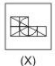SSC CPO Mock Test (Paper 1) - 7 - Question 46

In each question below is given a group of letters followed by four combinations of digits numbered (A), (B), (C) and (D). You have to find put which of the combinations correctly represents the group of letters based on the following coding system and mark the number of that combination as the answer. If none of the four combinations correctly represents the group of letters, mark (E) i.e. 'None of these' as the answer.
Letter : M Q I N E Y U G R
Digits :  2 5  3  7 1  8 4   6   9
Conditions:
(i) If the first and last letters are vowels both are to be coded as \$.
(ii) If the second letter is a vowel and the third letter is a consonant a single code is to be used and both are to be coded jointly as %.
(iii) If the first letter is a consonant and the last letter is a vowel both are to be coded as?

ENIMY

SSC CPO Mock Test (Paper 1) - 7 - Question 47

In each question below is given a group of letters followed by four combinations of digits numbered (A), (B), (C) and (D). You have to find put which of the combinations correctly represents the group of letters based on the following coding system and mark the number of that combination as the answer. If none of the four combinations correctly represents the group of letters, mark (E) i.e. 'None of these' as the answer.
Letter : M Q I N E Y U G R
Digits :  2 5  3  7 1  8 4   6   9
Conditions:
(i) If the first and last letters are vowels both are to be coded as \$.
(ii) If the second letter is a vowel and the third letter is a consonant a single code is to be used and both are to be coded jointly as %.
(iii) If the first letter is a consonant and the last letter is a vowel both are to be coded as?

GENIR

Detailed Solution for SSC CPO Mock Test (Paper 1) - 7 - Question 47

G → 6, E → coded as %, N → coded as % I → 3, R → 9 'EN' combined as % following the 2nd condition

SSC CPO Mock Test (Paper 1) - 7 - Question 48

Complete the analogous pair.

31. 11 : 145 :: 14 : ?

SSC CPO Mock Test (Paper 1) - 7 - Question 49

Select the correct mirror image of the figure (X) from amongst the given alternatives.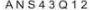SSC CPO Mock Test (Paper 1) - 7 - Question 50

Each of the following question consist of five problem figure marked 1, 2, 3, 4, 5 followed by Answer figure A, B, C, D and E. Select of figure from the answer figure which will continue the same series as given in the problem figure.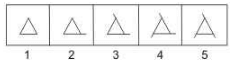SSC CPO Mock Test (Paper 1) - 7 - Question 51

In the questions, figure (X) is embedded in one of following figures. Find out the correct alternative.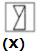SSC CPO Mock Test (Paper 1) - 7 - Question 52

Consider the following statements :
As per 2001 Census
1. The two states with the lowest sex ratio are Haryana and Punjab .
2. The two states with the lowest population per sq.km. of area are Meghalaya and Mizoram .
3. Kerala has both the highest literacy rate and sex ratio .
Which of the statements given above is/are correct ?

SSC CPO Mock Test (Paper 1) - 7 - Question 53

The nation state emerged -

SSC CPO Mock Test (Paper 1) - 7 - Question 54

Which amendment has included one more fundamental duty to Part-IV A?

SSC CPO Mock Test (Paper 1) - 7 - Question 55

Which of the following is not included in Article 19 of the Constitution?

SSC CPO Mock Test (Paper 1) - 7 - Question 56

The first indigenously built missile boat is named as

SSC CPO Mock Test (Paper 1) - 7 - Question 57

Consider the following statements about Internet facility:
I. It serves as the largest on-line information system.
II. It provides the most dependable and latest mode for sending E-mail.
III. Recurring expenditure on communicating E-mail is very high.
IV. It is often misused and has triggered cyber-crimes.
Which of these statements are correct?

SSC CPO Mock Test (Paper 1) - 7 - Question 58

There are ____________ bits in a byte.

SSC CPO Mock Test (Paper 1) - 7 - Question 59

Goodwill is a :

SSC CPO Mock Test (Paper 1) - 7 - Question 60

Which of the following is an example of credit investment?

SSC CPO Mock Test (Paper 1) - 7 - Question 61

The type of planning experimented in India between 1978 and 1980 is known as

SSC CPO Mock Test (Paper 1) - 7 - Question 62

Which is responsible to collect the service tax?

SSC CPO Mock Test (Paper 1) - 7 - Question 63

Which company will devlop the India's first washery reject coal-based power plant ?

SSC CPO Mock Test (Paper 1) - 7 - Question 64

The RBI Governor, in one of his speeches, made a mention of inflation index and said "The problem in India is that which inflation index to target as there are several such indices". Which of the following statements is CORRECT in this regard?

SSC CPO Mock Test (Paper 1) - 7 - Question 65

Production function express-

SSC CPO Mock Test (Paper 1) - 7 - Question 66

The Durgapur Steel Plant was set up with the aid and collaboration of

SSC CPO Mock Test (Paper 1) - 7 - Question 67

On which of the following river the Tulbul project is located ?

SSC CPO Mock Test (Paper 1) - 7 - Question 68

The countries with the largest population in descending order are :

SSC CPO Mock Test (Paper 1) - 7 - Question 69

The 'Milky way' Galaxy was first observed by—

SSC CPO Mock Test (Paper 1) - 7 - Question 70

Singareni Coal fields lie in -

SSC CPO Mock Test (Paper 1) - 7 - Question 71

Choose incorrect statement

SSC CPO Mock Test (Paper 1) - 7 - Question 72

Which one of the following is categorized as millet?

SSC CPO Mock Test (Paper 1) - 7 - Question 73

The Brihadeshwara temple at Thanjavur was built during the time of

SSC CPO Mock Test (Paper 1) - 7 - Question 74

Which among the following is not correctly matched?

SSC CPO Mock Test (Paper 1) - 7 - Question 75

Which of the following statements is not a fact ?

SSC CPO Mock Test (Paper 1) - 7 - Question 76

During Rigvedic period:

SSC CPO Mock Test (Paper 1) - 7 - Question 77

A 100kg man jumps into a swimming pool from a height of 5m. It takes 0.4 second for the water to reduce his velocity to zero. The average force exerted by water on the man, taking g=100m/s2, is

SSC CPO Mock Test (Paper 1) - 7 - Question 78

In which of the following states, the oil was first struck in 1889?

SSC CPO Mock Test (Paper 1) - 7 - Question 79

The 'stones' formed in human kidney consist mostly of

SSC CPO Mock Test (Paper 1) - 7 - Question 80

The chemical used as a 'fixed in photography is

SSC CPO Mock Test (Paper 1) - 7 - Question 81

If Safe storage is to be ensured, the moisture content of food grains at the time of harvesting should not be higher than

SSC CPO Mock Test (Paper 1) - 7 - Question 82

Which one of the following sets is correctly matched?

SSC CPO Mock Test (Paper 1) - 7 - Question 83

The major component of honey is

SSC CPO Mock Test (Paper 1) - 7 - Question 84

Which one of the following is used as an anti-freeze the automobile engines?

SSC CPO Mock Test (Paper 1) - 7 - Question 85

Most of the desert plants blooms during night time because

SSC CPO Mock Test (Paper 1) - 7 - Question 86

Which one of the following pairs of materials serves as electrodes in chargeable batteries commonly used in devices such as torch lights, electric shavers etc.?

SSC CPO Mock Test (Paper 1) - 7 - Question 87

India’s first radio festival has been orgainzed in which of the following cities?

SSC CPO Mock Test (Paper 1) - 7 - Question 88

Which animal fights snakes ?

SSC CPO Mock Test (Paper 1) - 7 - Question 89

Uranium is a naturally occurring radioactive element which emits alpha particles and is converted into

SSC CPO Mock Test (Paper 1) - 7 - Question 90

A ship floats on water. This can be explained by

SSC CPO Mock Test (Paper 1) - 7 - Question 91

Which metal is used for galvanising iron to immunize it against rusting ?

SSC CPO Mock Test (Paper 1) - 7 - Question 92

Distribution of electric power from one place to another is done by high AC voltage because :

SSC CPO Mock Test (Paper 1) - 7 - Question 93

The minimum possible temperature beyond which matter cannot be cooled is

SSC CPO Mock Test (Paper 1) - 7 - Question 94

If the density of water at 0ºC, 4ºC and 100ºC are denoted by X,Y and Z, then the correct sequence of their decreasing order is

SSC CPO Mock Test (Paper 1) - 7 - Question 95

Sleeping under a tree at night is dangerous since :

SSC CPO Mock Test (Paper 1) - 7 - Question 96

Which of the following polymers is widely used for making bullet proof material?

SSC CPO Mock Test (Paper 1) - 7 - Question 97

Which of the following is a set of five events included in Modern Pentathlon?

SSC CPO Mock Test (Paper 1) - 7 - Question 98

How many major organs does theU.N have?

SSC CPO Mock Test (Paper 1) - 7 - Question 99

Peat is the result of

SSC CPO Mock Test (Paper 1) - 7 - Question 100

The headquaters of 'Internaional Monetary Fund' are at:

SSC CPO Mock Test (Paper 1) - 7 - Question 101

Name the player to clinch the gold medal in 48 kg category at the National Women’s Boxing Championships held in Rohtak, Haryana

SSC CPO Mock Test (Paper 1) - 7 - Question 102

If α and β are actue angles and cos 2α=3 cos 2β-1/3-cos 2β, then tan α is equal to

SSC CPO Mock Test (Paper 1) - 7 - Question 103

A lad was asked his age by his friend. The lad said:"The number you get when you subtract 25 times my age from twice the square of my age will be thrice your age".If his friend's age will be 14,then the age of the lad is

Detailed Solution for SSC CPO Mock Test (Paper 1) - 7 - Question 103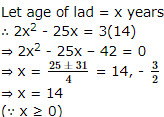SSC CPO Mock Test (Paper 1) - 7 - Question 104

What would be the maximum value of Q in the following equation?
5P9+3R7+2Q8=1114

Detailed Solution for SSC CPO Mock Test (Paper 1) - 7 - Question 104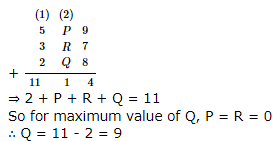SSC CPO Mock Test (Paper 1) - 7 - Question 105

A table costs Rs 1,600 more than a chair.If the total cost of a table and a chair is Rs 2,800,what is the cost of a chair?

SSC CPO Mock Test (Paper 1) - 7 - Question 106

If 4x + 3y = 18xy and 2x - 5y + 4xy = 0 then the values of x and y will be respectively

Detailed Solution for SSC CPO Mock Test (Paper 1) - 7 - Question 106

4x + 3y = 18xy .....(1)
2x - 5y + 4xy = 0 .....(2)
Multiplying 2 in equation (2) we get
2 (2x - 5y + 4xy) = 0
⇒ 4x - 10y + 8xy = 0
⇒ 4x - 10y = - 8xy .....(3)
Subtracting equation (3) from equation (1) we get
13y = 26xy
⇒ x = 1 2
Putting the value of x in equation (1) we get
4 ( 1 2 ) + 3y = 18 ( 1 2 ) y
⇒ 2 + 3y = 9y
⇒ 2 = 6y
⇒ y = 1 3

SSC CPO Mock Test (Paper 1) - 7 - Question 107

The area of the sector of the circle is 1/10th the area of the circle. The angle of the sector is

Detailed Solution for SSC CPO Mock Test (Paper 1) - 7 - Question 107

Area of circle = A = πr2
Area of sectors of circle A = n 360 πr2
Where n = angle of the sector
According to question
n 360 πr2 = πr2 x 1 10
⇒ n 360 = 1 10
⇒ 10n = 360
⇒ n = 360 10 = 36

SSC CPO Mock Test (Paper 1) - 7 - Question 108

The solution of 2x+3 / 2x-1=3x-1 / 3x+1 is

SSC CPO Mock Test (Paper 1) - 7 - Question 109

The area of the quadrant of a circle whose circumference is 22 cm is

Detailed Solution for SSC CPO Mock Test (Paper 1) - 7 - Question 109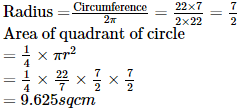SSC CPO Mock Test (Paper 1) - 7 - Question 110

By which smallest number must 1323 be multiplied so that it becomes a perfect cube?

SSC CPO Mock Test (Paper 1) - 7 - Question 111

If the coordinates of A, B, C are (4,4), (3,-2) and (3,-16), then the area of triangle is

SSC CPO Mock Test (Paper 1) - 7 - Question 112

The average salary of male employees in a firm was Rs 5200 and that of females was Rs 4200. The mean salary of all the employees was Rs 5000,Percentage of female employees is

Detailed Solution for SSC CPO Mock Test (Paper 1) - 7 - Question 112

Let total number of employees = 100
Let total number of female employees = x
∴ Male employees = 100 - x
so 5200 (100 - x) + 4200x 100 = 5000
or 520000 - 5200x + 4200x = 50,0000
or 20000 = 1000x
∴ x = 20
Hence, percentage of female employees = 20 100 x 100 = 20%

SSC CPO Mock Test (Paper 1) - 7 - Question 113

There is a circular pond and a foot-path runs along its boundary. A man walks around it exactly once, keeping close to the edge. His each step is 55 cm long and he takes exactly 440 steps to around once then. The diameter of the pond is

Detailed Solution for SSC CPO Mock Test (Paper 1) - 7 - Question 113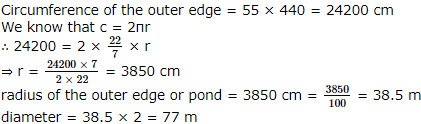SSC CPO Mock Test (Paper 1) - 7 - Question 114

A sum of money doubles itself in 4 years at compound interest. It will amount to 8 times itself at the same rate of interest in

Detailed Solution for SSC CPO Mock Test (Paper 1) - 7 - Question 114

Let the principal amount be P and rate of interest be R% time taken, t1 = 4 year
When, amount becomes double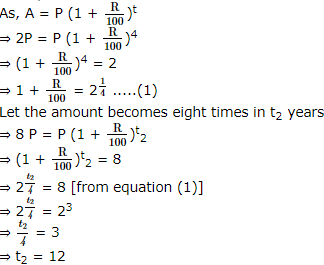SSC CPO Mock Test (Paper 1) - 7 - Question 115

Three math classes : X, Y and Z take an algebra test
1. The average score of class X is 83
2. The average score of class Y is 76.
3. The average score of class Z is 85.
4. Average score of class X and Y is 79.
5. Average score of class Y and Z is 81.
What is the average score of classes X, Y, Z?

Detailed Solution for SSC CPO Mock Test (Paper 1) - 7 - Question 115

Let the number of students in classes X, Y and Z be a, b and c respectively.
Then, Total score of X = 83; Total score of Y = 76b
Total score of Z = 85c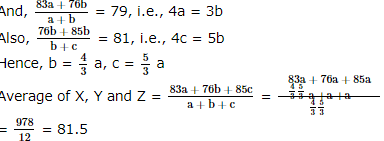SSC CPO Mock Test (Paper 1) - 7 - Question 116

1 + 1/5 + 1/52 + 1/53 = ?

SSC CPO Mock Test (Paper 1) - 7 - Question 117

D, E and F are the mid-points of sides of an equilateral triangle ABC, then the ratio of the area of triangles DEF and DCE is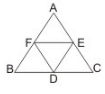Detailed Solution for SSC CPO Mock Test (Paper 1) - 7 - Question 117

Δ P E F ≅ Δ D C E ⇒

they are equal in area.

SSC CPO Mock Test (Paper 1) - 7 - Question 118

A shopkeeper marks his goods at 40% above the cost price and allows a discount of 40% on the marked price. His loss or gain is

SSC CPO Mock Test (Paper 1) - 7 - Question 119

If PT is a tangent and PAB is a secant to a circle from a external point P. If PT = 8 cm and PA = 16 cm then length of AB is

Detailed Solution for SSC CPO Mock Test (Paper 1) - 7 - Question 119

Use PT2 = PA x PB

SSC CPO Mock Test (Paper 1) - 7 - Question 120

An angle is one fifth of its supplement. The measure of the angle is

Detailed Solution for SSC CPO Mock Test (Paper 1) - 7 - Question 120

x = ( 1 5 ) (180-x)
x = 30

SSC CPO Mock Test (Paper 1) - 7 - Question 121

D and E are the mid-point of sides BC and CA or ∆ABC. AD and BE meet at O. If area ∆ABC = 60 square units then area ∆AOB is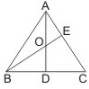Detailed Solution for SSC CPO Mock Test (Paper 1) - 7 - Question 121

AD and BE are medians. Clearly a r . Δ A O B = 1 3 a r . Δ A B C

SSC CPO Mock Test (Paper 1) - 7 - Question 122

Of the three numbers,the first is 3 times the second and the third is 5 times the first.If the average of the three numbers is 57,then the difference between the largest and the smallest number is

SSC CPO Mock Test (Paper 1) - 7 - Question 123

What approximate value should come in the place of the question mark in the following equation? 3 3/5 of 157.85 + 39% of 1847 = ?-447.30

SSC CPO Mock Test (Paper 1) - 7 - Question 124

If two numbers are in the ratio 6:13 and their least common multiple is 312,then the sum of the numbers is

SSC CPO Mock Test (Paper 1) - 7 - Question 125

The length of the shadow of a vertical pole of height h, thrown by the sun's rays at three different moments are h, 2h and 3h. The sum of the angles of elevation of the rays at these three moments is equal to

SSC CPO Mock Test (Paper 1) - 7 - Question 126

A water tank is 2/ 5 th full. Pipe A can fill the tank in 10 minutes and the pipe B can empty it in 6 minutes. If both the pipes are open, how long will it take to empty or fill the tank completely?

Detailed Solution for SSC CPO Mock Test (Paper 1) - 7 - Question 126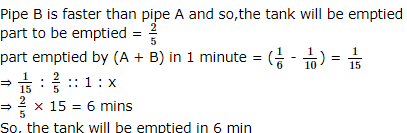SSC CPO Mock Test (Paper 1) - 7 - Question 127

Aditya invests 60% of his total investment in machinery,25% of the remaining in raw material and now has Rs 15,000 cash with him. His total investment is

Detailed Solution for SSC CPO Mock Test (Paper 1) - 7 - Question 127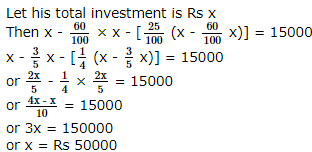SSC CPO Mock Test (Paper 1) - 7 - Question 128

A discount of 15% on one article is the same as a discount of 20% on another article. The costs of the two articles can be:

Detailed Solution for SSC CPO Mock Test (Paper 1) - 7 - Question 128

Let the costs of the two articles be x and y.
Then 15% of x = 20% of y
⇒ X y = 20 15 = 4 3
so x and y must be in the ratio of 4 : 3

SSC CPO Mock Test (Paper 1) - 7 - Question 129

If in any ∆ABC, a=(√3+1)cm and ∠B=30º, ∠C=45º,, then the area of triangle is

SSC CPO Mock Test (Paper 1) - 7 - Question 130

Profit after selling an article for Rs 425 is the same as the loss after selling it for Rs 355. The cost of the article is

Detailed Solution for SSC CPO Mock Test (Paper 1) - 7 - Question 130

Let c.p. = Rs x
∴ 425 - x = x - 355
⇒ 2x = 425 + 355 = 780
⇒ x = 780 2 = Rs 390

SSC CPO Mock Test (Paper 1) - 7 - Question 131

If a : b = 2 : 3 and b : c = 4 : 5, find a2 : b2 : c2

Detailed Solution for SSC CPO Mock Test (Paper 1) - 7 - Question 131

a : b = 2 : 3
⇒ a2 : b2 = 4 : 9 .....(1)
Also,
b : c = 4 : 5
⇒ b2 : c2 = 16 : 25 .....(2)
Combining (1) and (2)
a2 : b2 : c2 = 64 : 144 : 225

SSC CPO Mock Test (Paper 1) - 7 - Question 132

Two numbers are in the ratio 4:5. If each is diminished by 6,then they would be in the ratio 3:4.Find the pair of numbers.

SSC CPO Mock Test (Paper 1) - 7 - Question 133

pth term of an A.P. is q and qth term is P,its rth term is

SSC CPO Mock Test (Paper 1) - 7 - Question 134

If 5 persons can do 5 times of a work in 5 days, then 10 persons can do 10 times of that work in

SSC CPO Mock Test (Paper 1) - 7 - Question 135

Mr. Roy borrowed some money from Mr. Das. The rate of interest for first two years is 8% per annum, for the next three years is 11% per annum, for the period beyond 5 Years is 14% per annum. Mr. Das got an amount of Rs. 10920 as an interest at the end of eight years. The what amount was borrowed by Mr. Roy ?

SSC CPO Mock Test (Paper 1) - 7 - Question 136

What should come in the place of both the (?) in the following equation? 175/? = ?/63

SSC CPO Mock Test (Paper 1) - 7 - Question 137

A man climbs a hill at a speed of 1 metre/second and returns to ground at twice the speed. His average speed for the round trip is

SSC CPO Mock Test (Paper 1) - 7 - Question 138

If sinA+sinB=C, then cosA+cosB=D, then sin(A+B)=

SSC CPO Mock Test (Paper 1) - 7 - Question 139

[(sin3θ+sin5θ+sin7θ+sin9θ)/(cos3θ+cos5θ+cos7θ+cos9θ)]=

SSC CPO Mock Test (Paper 1) - 7 - Question 140

A sphere of radius 3 cm is dropped into a cylindrical vessel partly filled with water. The radius of the vessel is 6 cm. If the sphere is submerged completely, the surface of the water is raised by

SSC CPO Mock Test (Paper 1) - 7 - Question 141

If the radius of the base and the height of a right circular cone are doubled, then its volume becomes:

Detailed Solution for SSC CPO Mock Test (Paper 1) - 7 - Question 141

Let the original radius and height of the cone be r and h respectively.
Then, new radius = 2r. New height = 2h
∴ New Volume Original Volume = 1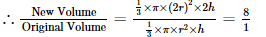SSC CPO Mock Test (Paper 1) - 7 - Question 142

A spherical ball made of iron has diameter 6 cm. If density of iron is 8 g/cm3 than mass of the ball is nearly (Use π = 3.142)

SSC CPO Mock Test (Paper 1) - 7 - Question 143

Study the following graph carefully to answer these questions.
Quantity of various items sold and price per kg.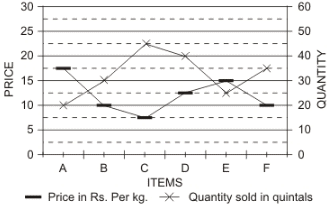If the quantity sold of item D increased by 50% and the price reduced by 10%, what was the total value of the quantity sold for item D ?

Detailed Solution for SSC CPO Mock Test (Paper 1) - 7 - Question 143

New quantity of item D = 40 ×150/100 = 60 quintal New price/kg of item D = 90 % of Rs. 12.5 = 12.5 ×90/100 = Rs. 11.25/kg ∴ Total price = Rs. (60 × 100 × 11.25) = Rs. 67500

SSC CPO Mock Test (Paper 1) - 7 - Question 144

Study the following graph carefully to answer these questions.
Quantity of various items sold and price per kg.Approximately, what is the average price per kg. of items A,B and C ?

SSC CPO Mock Test (Paper 1) - 7 - Question 145

Study the following graph carefully to answer these questions.
Quantity of various items sold and price per kg.What is the ratio between the total values of quantity sold for items E and F respectively ?

SSC CPO Mock Test (Paper 1) - 7 - Question 146

Study the following graph carefully to answer these questions.
Quantity of various items sold and price per kg.Total value of the quantity sold for item C is what percent of the total value of the quantity sold for item E ?

SSC CPO Mock Test (Paper 1) - 7 - Question 147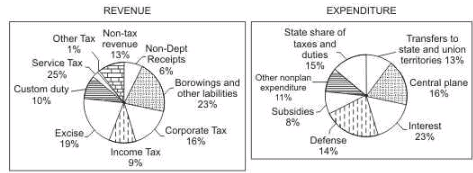The pie charts show estimated revenue and expenditure budgets for 2004-2005 for country X.
Notes: 1. Corporate tax revenues increased by Rs.2545 crore in the budget estimates for 2004-2005 over the estimates for 2003-2004.
2. Service tax brings in Rs.3990crore according to the budget for 2004-2005.
3. Total expenditure on defence increased by a whopping 28 percent from Rs.6030crore in 2003-2004 to Rs.7700crore in 2004-2005.

The revenue generated by Custom duty, Income tax and Corporate tax according to the budget for 2004-2005 is.

SSC CPO Mock Test (Paper 1) - 7 - Question 148The pie charts show estimated revenue and expenditure budgets for 2004-2005 for country X.
Notes: 1. Corporate tax revenues increased by Rs.2545 crore in the budget estimates for 2004-2005 over the estimates for 2003-2004.
2. Service tax brings in Rs.3990crore according to the budget for 2004-2005.
3. Total expenditure on defence increased by a whopping 28 percent from Rs.6030crore in 2003-2004 to Rs.7700crore in 2004-2005.

What is the percentage increase in the revenue generated by corporate tax is 2004-2005 over 2003-2004 according to the budgets?

SSC CPO Mock Test (Paper 1) - 7 - Question 149The pie charts show estimated revenue and expenditure budgets for 2004-2005 for country X.
Notes: 1. Corporate tax revenues increased by Rs.2545 crore in the budget estimates for 2004-2005 over the estimates for 2003-2004.
2. Service tax brings in Rs.3990crore according to the budget for 2004-2005.
3. Total expenditure on defence increased by a whopping 28 percent from Rs.6030crore in 2003-2004 to Rs.7700crore in 2004-2005.

If the percentage distribution for the sectors from he Expenditure graph remain the same for the 2003-2004 and 2004-2005 budgets, then what is the difference in budgeted expenditures in 2003-2004 and 2004-2005?

SSC CPO Mock Test (Paper 1) - 7 - Question 150The pie charts show estimated revenue and expenditure budgets for 2004-2005 for country X.
Notes: 1. Corporate tax revenues increased by Rs.2545 crore in the budget estimates for 2004-2005 over the estimates for 2003-2004.
2. Service tax brings in Rs.3990crore according to the budget for 2004-2005.
3. Total expenditure on defence increased by a whopping 28 percent from Rs.6030crore in 2003-2004 to Rs.7700crore in 2004-2005.

According to the budget for 2004-2005, the revenue generated by non-debt capital receipts is what percent of expenditure on subsides?

SSC CPO Mock Test (Paper 1) - 7 - Question 151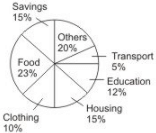Above circle graph shows the percent of money spent by family on various items during 1999. Study the graph and answer these questions.

If the total amount spent during the year 1999 was Rs. 46000, the amount spent on food was:

SSC CPO Mock Test (Paper 1) - 7 - Question 152

If 'spoon' is called 'plate', 'plate' is called knife', 'knife' is called 'jug', 'jug' is called 'glass', 'glass' is called 'saucer' and 'saucer' is called 'spoon', by what do we cut fruit?

SSC CPO Mock Test (Paper 1) - 7 - Question 153

Find the Antonym of FOLLOWING

SSC CPO Mock Test (Paper 1) - 7 - Question 154

Find the antonym of APPOSITE

SSC CPO Mock Test (Paper 1) - 7 - Question 155

Choose the correctly spelt word.

SSC CPO Mock Test (Paper 1) - 7 - Question 156

Find the Antonym of OPTIONAL

SSC CPO Mock Test (Paper 1) - 7 - Question 157

Find the Antonym of FACT

SSC CPO Mock Test (Paper 1) - 7 - Question 158

Choose the correctly spelt word.

SSC CPO Mock Test (Paper 1) - 7 - Question 159

Find the Antonym of SUBSERVIENT

SSC CPO Mock Test (Paper 1) - 7 - Question 160

Choose the correctly spelt word.

SSC CPO Mock Test (Paper 1) - 7 - Question 161

Fill in the blank with appropriate word.
Because of diesel........many garages are introducing rationing.

SSC CPO Mock Test (Paper 1) - 7 - Question 162

Fill in the blank with appropriate word.
Jessica Mitford wrote "The American Way of Death", a best-selling book, ......to an official investigation of the funeral industry.

SSC CPO Mock Test (Paper 1) - 7 - Question 163

Choose the correctly spelt word.

SSC CPO Mock Test (Paper 1) - 7 - Question 164

Choose the correctly spelt word.

SSC CPO Mock Test (Paper 1) - 7 - Question 165

Fill in the blank with appropriate word.
Since the road was closed for repairs he took the........

SSC CPO Mock Test (Paper 1) - 7 - Question 166

Choose the one which can be substituted for the given words/sentences
A person who insists on something.

SSC CPO Mock Test (Paper 1) - 7 - Question 167

Choose the one which can be substituted for the given words/sentences
Habitually silent or talking little.